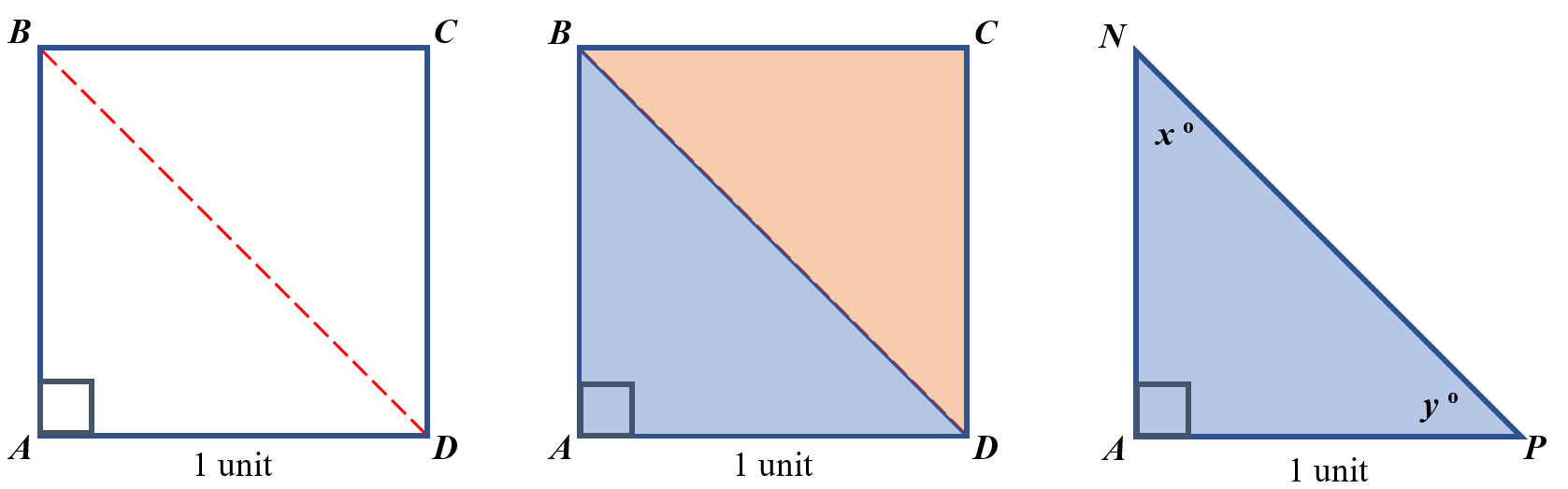# GEOM 2 | Lesson 3 | Try This! (Special Right Triangles #1)# Special Right Triangles #1

Two congruent triangles can be formed by folding a square with sides = 1 unit on either of its diagonals as shown in $$\square ABCD$$.  One of those triangles formed is labeled $$\triangle PAN$$.1. What are the lengths of the legs of $$\triangle PAN$$?
2. Use the Pythagorean theorem to find the length of the hypotenuse $$\overline{\rm PN}$$.
3. What are the measurements of angles $$x$$ and $$y$$?
4. What type of triangle is $$\triangle PAN$$?
5. Sketch a new triangle that is similar to $$\triangle PAN$$ with legs that measure 2 units each.  Note: Similar triangles have congruent corresponding angles.
6. What is the length the hypotenuse for this new triangle (be sure to simplify the square root) ?
7. How do the lengths of the legs compare to the length of the hypotenuse?
8. Is this relationship true for any triangle that is similar to $$\triangle PAN$$?